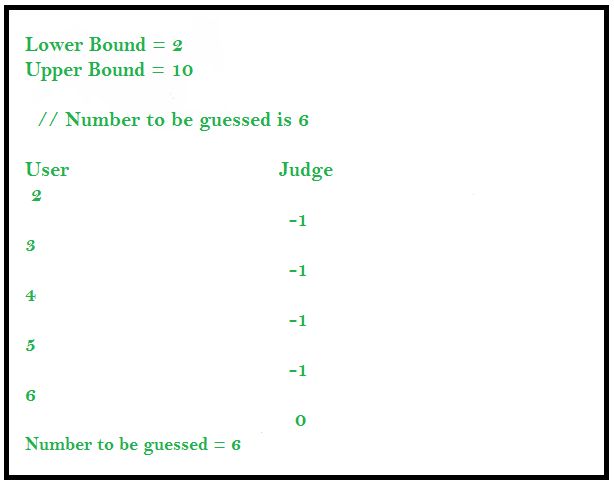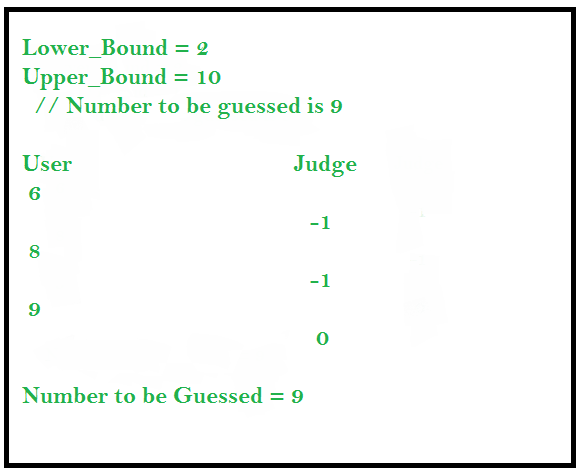# Interactive Problems in Competitive Programming

• Difficulty Level : Easy
• Last Updated : 23 May, 2022

Interactive Problems are those problems in which our solution or code interacts with the judge in real time. When we develop a solution for an Interactive Problem then the input data given to our solution may not be predetermined but is built for that problem specifically. The solution performs a series of exchange of data with the judge and at the end of the conversation the judge decides whether our solution was correct or not.

## Guessing the Number (An Interactive Problem)

In this problem the user has to guess the number during a communication with the judge. The user is provided with the upper and lower bound and he/she can ask the judge whether a number is the number to be guessed. The judge replies with -1 if the number is smaller than the number to be guessed or 1 if number is greater than the number to be guessed or 0 if it is equal to the number to be guessed.

### Approach 1 : Linear Guessing

The user can query the judge for all the numbers between lower limit and upper limit to find the solution.## C++

 `#include ` `using` `namespace` `std;`   `int` `main()` `{` `    ``int` `lower_bound = 2; ` `    ``int` `upper_bound = 10; `   `    ``// Number to be guessed is 6 `   `    ``// Iterating from lower_bound to upper_bound ` `    ``for` `(``int` `i = lower_bound; i <= upper_bound; i++) { ` `        ``cout << i << endl; `   `        ``// Input the response from the judge ` `        ``int` `response;` `        ``cin >> response; `   `        ``if` `(response == 0) { ` `            ``cout << ``"Number guessed is :"` `<< i; ` `            ``break``; ` `        ``} ` `    ``} `   `    ``return` `0;` `}`   `// This code is contributed by divyeshrabadiya07`

## Java

 `import` `java.util.*;` `class` `GFG {` `    ``public` `static` `void` `main(String[] args)` `    ``{` `        ``Scanner sc1 = ``new` `Scanner(System.in);` `        ``int` `lower_bound = ``2``;` `        ``int` `upper_bound = ``10``;`   `        ``// Number to be guessed is 6`   `        ``// Iterating from lower_bound to upper_bound` `        ``for` `(``int` `i = lower_bound; i <= upper_bound; i++) {` `            ``System.out.println(i);`   `            ``// Input the response from the judge` `            ``int` `response = sc1.nextInt();`   `            ``if` `(response == ``0``) {` `                ``System.out.println(``"Number guessed is :"` `+ i);` `                ``break``;` `            ``}` `        ``}` `    ``}` `}`

## Python3

 `if` `__name__``=``=``'__main__'``:` `    ``lower_bound ``=` `2``; ` `    ``upper_bound ``=` `10``; `   `    ``# Number to be guessed is 6 `   `    ``# Iterating from lower_bound to upper_bound ` `    ``for` `i ``in` `range``(lower_bound, upper_bound ``+` `1``):` `        ``print``(i)`   `        ``# Input the response from the judge ` `        ``response ``=` `int``(``input``())`   `        ``if` `(response ``=``=` `0``):` `            ``print``(``"Number guessed is :"``, i, end ``=` `'') ` `            ``break``; `   `            ``# This code is contributed by rutvik_56`

## C#

 `using` `System;` `class` `GFG` `{` `    ``public` `static` `void` `Main(``string``[] args)` `    ``{       ` `        ``int` `lower_bound = 2;` `        ``int` `upper_bound = 10;` ` `  `        ``// Number to be guessed is 6` ` `  `        ``// Iterating from lower_bound to upper_bound` `        ``for` `(``int` `i = lower_bound; i <= upper_bound; i++) ` `        ``{` `            ``Console.WriteLine(i);` ` `  `            ``// Input the response from the judge` `            ``int` `response = ``int``.Parse(Console.ReadLine());` ` `  `            ``if` `(response == 0) {` `                ``Console.WriteLine(``"Number guessed is :"` `+ i);` `                ``break``;` `            ``}` `        ``}` `    ``}` `}`   `// This code is contributed by Pratham76`

Output

```2
Number guessed is :2```

Time Complexity: O(n)

### Approach 2 : Applying Binary Search

We can also apply binary search interactively to find the solution. This solution is efficient as compared to the previous approach.## C++

 `#include `   `using` `namespace` `std;`   `int` `main(``int` `argc, ``char``** argv)` `{` `    ``int` `lower_bound = 2;` `    ``int` `upper_bound = 10;`   `    ``while` `(lower_bound <= upper_bound)` `    ``{` `        ``int` `mid = (lower_bound + upper_bound) / 2;` `        ``cout << mid << endl;` `        ``int` `response;` `        ``cin >> response;`   `        ``if` `(response == -1)` `            ``lower_bound = mid + 1;`   `        ``else` `if` `(response == 1)` `            ``upper_bound = mid - 1;` `        `  `        ``else` `if` `(response == 0){` `            ``cout << ``"Number guessed is :"` `+ to_string(mid) << endl;` `            ``break``;` `        ``}` `    ``}` `    ``return` `0;` `}`   `// This code is contributed by geeky01adarsh`

## Java

 `import` `java.util.*;` `class` `GFG {` `    ``public` `static` `void` `main(String[] args)` `    ``{` `        ``Scanner sc1 = ``new` `Scanner(System.in);` `        ``int` `lower_bound = ``2``;` `        ``int` `upper_bound = ``10``;`   `        ``// Number to be guessed is 9`   `        ``// Applying Binary Search interactively` `        ``while` `(lower_bound <= upper_bound) {` `            ``int` `mid = (lower_bound + upper_bound) / ``2``;`   `            ``// Print the guessed number` `            ``System.out.println(mid);`   `            ``// Input the response from the judge` `            ``int` `response = sc1.nextInt();`   `            ``if` `(response == -``1``) {` `                ``lower_bound = mid + ``1``;` `            ``}` `            ``else` `if` `(response == ``1``) {` `                ``upper_bound = mid - ``1``;` `            ``}` `            ``else` `if` `(response == ``0``) {` `                ``System.out.println(``"Number guessed is :"` `+ mid);` `                ``break``;` `            ``}` `        ``}` `    ``}` `}`

## C#

 `using` `System;` `class` `GFG {` `  ``static` `void` `Main() {` `    ``int` `lower_bound = 2;` `    ``int` `upper_bound = 10;`   `    ``// Number to be guessed is 9`   `    ``// Applying Binary Search interactively` `    ``while` `(lower_bound <= upper_bound) {` `        ``int` `mid = (lower_bound + upper_bound) / 2;`   `        ``// Print the guessed number` `        ``Console.WriteLine(mid);`   `        ``// Input the response from the judge` `        ``int` `response = Convert.ToInt32(Console.ReadLine());`   `        ``if` `(response == -1) {` `            ``lower_bound = mid + 1;` `        ``}` `        ``else` `if` `(response == 1) {` `            ``upper_bound = mid - 1;` `        ``}` `        ``else` `if` `(response == 0) {` `            ``Console.WriteLine(``"Number guessed is :"` `+ mid);` `            ``break``;` `        ``}` `    ``}` `  ``}` `}`   `// This code is contributed by divyesh072019`

## Python3

 `lower_bound ``=` `2` `upper_bound ``=` `10`   `# Number to be guessed is 9`   `# Applying Binary Search interactively` `while` `(lower_bound <``=` `upper_bound) :` `    ``mid ``=` `(lower_bound ``+` `upper_bound) ``/``/` `2`   `    ``# Print guessed number` `    ``print``(mid)`   `    ``# Input the response from the judge` `    ``response ``=` `int``(``input``())`   `    ``if` `(response ``=``=` `-``1``) :` `        ``lower_bound ``=` `mid ``+` `1` `    `  `    ``elif` `(response ``=``=` `1``) :` `        ``upper_bound ``=` `mid ``-` `1` `    `  `    ``elif` `(response ``=``=` `0``) :` `        ``print``(``"Number guessed is :"``, mid)` `        ``break`

Output

```6
Number guessed is :6```

Time Complexity: O(logn)
Algorithm Paradigm: Divide and Conquer

My Personal Notes arrow_drop_up
Recommended Articles
Page :